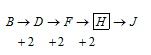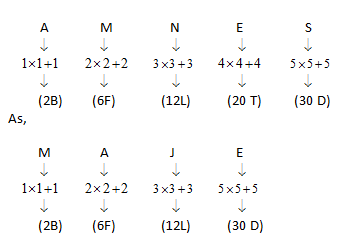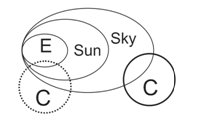# SSC CGL EXAMS 2019 | Reasoning Ability Practice Questions (Day-21)

Dear Aspirants, Here we have given the Important SSC Exam 2019 Practice Test Papers. Candidates those who are preparing for SSC 2019 can practice these questions to get more confidence to Crack SSC 2019 Examination.

[WpProQuiz 4859]

Find the missing number of the following questions

1) 1, 1, 16,?, 361, 132496.

a) 561

b) 271

c) 361

d) 400

2) B58, D41, F71,?, J99

a) H69

b) H96

c) H216

d) H612

3) Pine apple’ is related to soil is the same way as ‘kiwi’ is related to—

a) Red – soil

b) Air

c) Satellite

d) None of these

4) ‘10’ is related to ‘pole’ is the same way ‘O’ is related to.

a) Earth surface

b) Center of Earth

c) Out of core surface of Earth

d) None of these

5) Find the odd one out from the given alternatives.

a) Frog

b) Snake

c) Insects

d) Deer

6) AMNES’ is writer as ‘BFLTD’ in a certain code language, then ‘MAJE’ is written as on the same way?

a) BFLD

b) FBDL

c) NJZAE

d) None of these

7) In each of the following question below are given some statements followed by some conclusions. Taking the given statements to be true even if they seem to be at variance from commonly known facts, read all the conclusions and then decide which of the given conclusion logically follows the given statements.

Statement I.    a≥b,     b > c

Statement II.  d≤c,    e  > d

Conclusions:

a > c

b > d

a) Only I is true

b) Only II is true

c) Both true

d) None of these

8) In each of the following question below are given some statements followed by some conclusions. Taking the given statements to be true even if they seem to be at variance from commonly known facts, read all the conclusions and then decide which of the given conclusion logically follows the given statements.

Statement I. All ‘Earth’ is sun

Statement II. All sun is sky.

Statement III. Some sky is cloud.

Conclusions: I) All Sky is Earth

II) Some Sun is cloud

a) Only III is true

b) Only II is true

c) Either I or II is true

d) Both I is II is true.

9) In each of the following question below are given some statements followed by some

conclusions. Taking the given statements to be true even if they seem to be at variance from commonly known facts, read all the conclusions and then decide which of the given conclusion logically follows the given statements.

P \$ Q means; P is brother of Q.

P © Q means; P is Father of Q.

P * Q means; P is sister of Q.

P @ Q means; P is mother of Q.

A © B @ C * D \$ E

a) Maternal grand father

b) Maternal grand mother

c) Father

d) Mother

10) Complete the following series in a certain logical way-

DFFD100, EBCD49, BEGC?

a) 100

b) 729

c) 900

d) 121

1| (1 + 3)2 = 16| (16 + 3)2 = 361| (361 + 3)2 = 132496&53 = 125 = 8 (1+2+5)

43 = 64 = 10 = 1 (1+0)

73 = 343 = 10 = 1 (1+0)

63 = 216 = 9 (2+1+6)

Pineapple is a fruit which grows in soil. Kiwi is a fruit which grows is air.

10   Pole, because, the value of ‘g’ of a pole is maximum which is 10. In the same way ‘O’ is the value of ‘g’ at the center of Earth.

Deer is animal which is vegetarian. Rest of all the three options, insects, frog & snake are forming a group where Frog eat to insects, and the snake eats to frog and this is called carnivore in Biology.By both the statement,

a ≥ b > c ≥ d < e from above logical arrangement,

= a > c & b > d are true.According the above diagram conclusion I is true because it is different case and conclusion II is not true because it is indefinite case.

A is father of B, B is mother C, C is sister of D, D is brother of E. So, A is maternal grandfather of E.

DFFD = 4+6×6+4=102 = 100, EBCD = 5+2 = 7×7 = 49,

BEGC = 2+5×7+3 = 102 = 100

*********************************